# Can you describe how to get the answer A picture is hanging from the two ropes...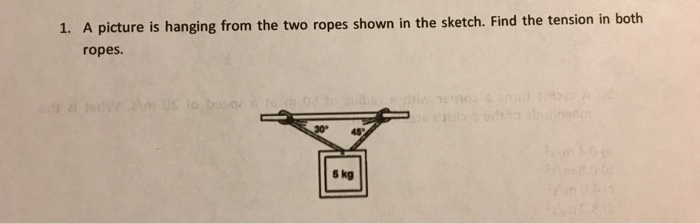Can you describe how to get the answer
A picture is hanging from the two ropes shown in the sketch. Find the tension in both ropes.

left one be T1 and right be T2

resolving them into components

T1 cos30 = T2 cos45 [these are the horizontal forces]

T1 = 0.816*T2

T1*sin30 + T2*sin45 = 5*9.81 = 49.05

T1/2 + T2/root(2) = 49.05

0.408 T2 + 0.707*T2 = 49.05

T2 = 43.98 N

T1 = 0.816*43.98 = 35.89 N

#### Earn Coin

Coins can be redeemed for fabulous gifts.

Similar Homework Help Questions
• ### An unknown mass is hanging by a cable which is held by two ropes anchored to...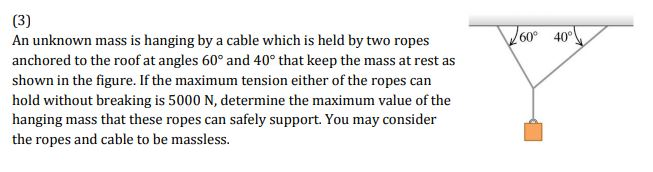An unknown mass is hanging by a cable which is held by two ropes anchored to the roof at angles 60° and 40° that keep the mass at rest as shown in the figure. If the maximum tension either of the ropes can hold without breaking is 5000 N, determine the maximum value of the hanging mass that these ropes can safely support. You may consider the ropes and cable to be massless 60 40° An unknown mass is hanging...

• ### 60° 40° An unknown mass is hanging by a cable which is held by two ropes...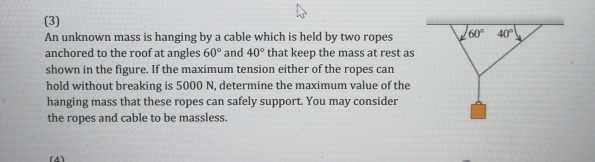60° 40° An unknown mass is hanging by a cable which is held by two ropes anchored to the roof at angles 60° and 40° that keep the mass at rest as shown in the figure. If the maximum tension either of the ropes can hold without breaking is 5000 N, determine the maximum value of the hanging mass that these ropes can safely support. You may consider the ropes and cable to be massless.

• ### A 770N acrobat is hanging at rest from two ropes as shown. Hint: It may be helpful to work in terms of Ty for this...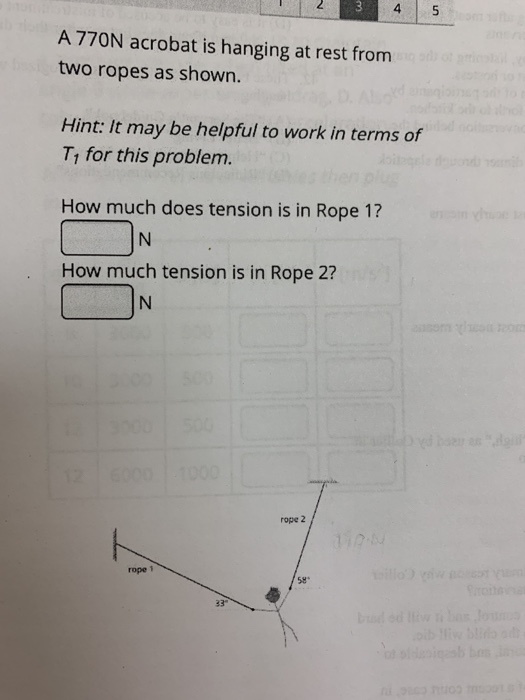A 770N acrobat is hanging at rest from two ropes as shown. Hint: It may be helpful to work in terms of Ty for this problem. How much does tension is in Rope 1? IN How much tension is in Rope 2? N rope 2

• ### tension of two ropes

Two angled ropes used to support the crate can withstand a maximum tension of 1500N. What is the largest mass the ropes can support? (Ans: 170kg) How do you get tothat answer??http://session.masteringphysics.com/problemAsset/1176891/2/ch05.P007.jpg

• ### S A lamp is hanging from a ceiling by two ropes. One rope makes an angle...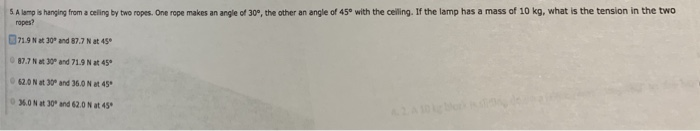S A lamp is hanging from a ceiling by two ropes. One rope makes an angle of 30P, the other an angle of 45° with the ceiling. If the lamp has a mass of 10 kg, what is the tension in the two ropes? 71.9 N at 30 and 87.7 N at 45 87.7 N at 30 and 71.9 N at 45 62.0N at 30 and 36.0N et 45 36.0 N at 30 and 62.0 N at 45

• ### Hanging weight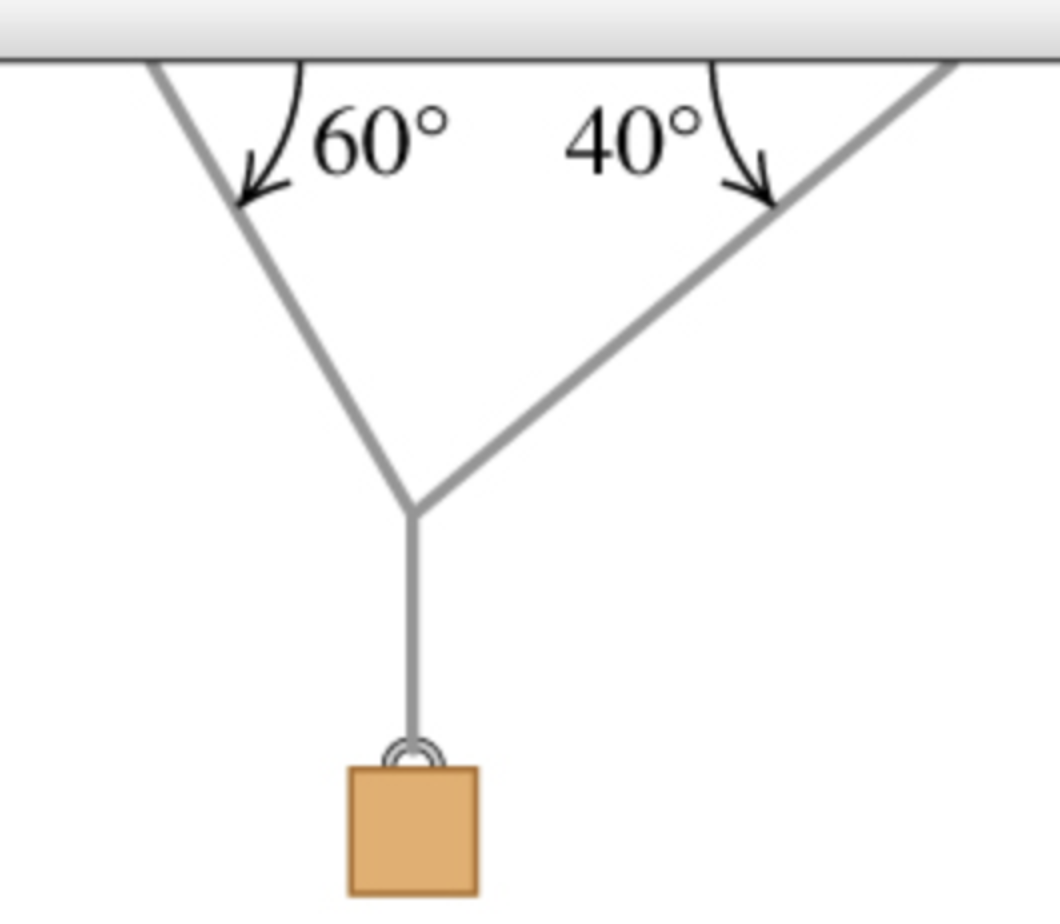Two ropes are connected to a steel cable that supports a hanging weight as shown in the figure.1) Draw a free-body diagram showing all of the forces acting at the knot that connects the two ropes to the steel cable.Draw the force vectors with their tails at the knot. The orientation of your vectors will be graded. The exact length of your vectors will not be graded but the relative length of one to the other will be graded.2) Based...

• ### A person walks into an elevator holding two masses hanging on ropes, one below the other....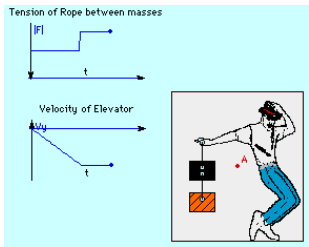A person walks into an elevator holding two masses hanging on ropes, one below the other. The top mass is 6.35 kg and the lower mass is 2.28 kg. The elevator begins to move downward with an acceleration of 5.04 m/s/s before reaching a constant velocity. (a) How much tension (in Newtons) is in the rope between the masses during the acceleration? (b) If the elevator cable suddenly broke and the elevator were in freefall, what would the lower hanging...

• ### Part A A 500 kg steel beam is supported by the two ropes shown in (Figure...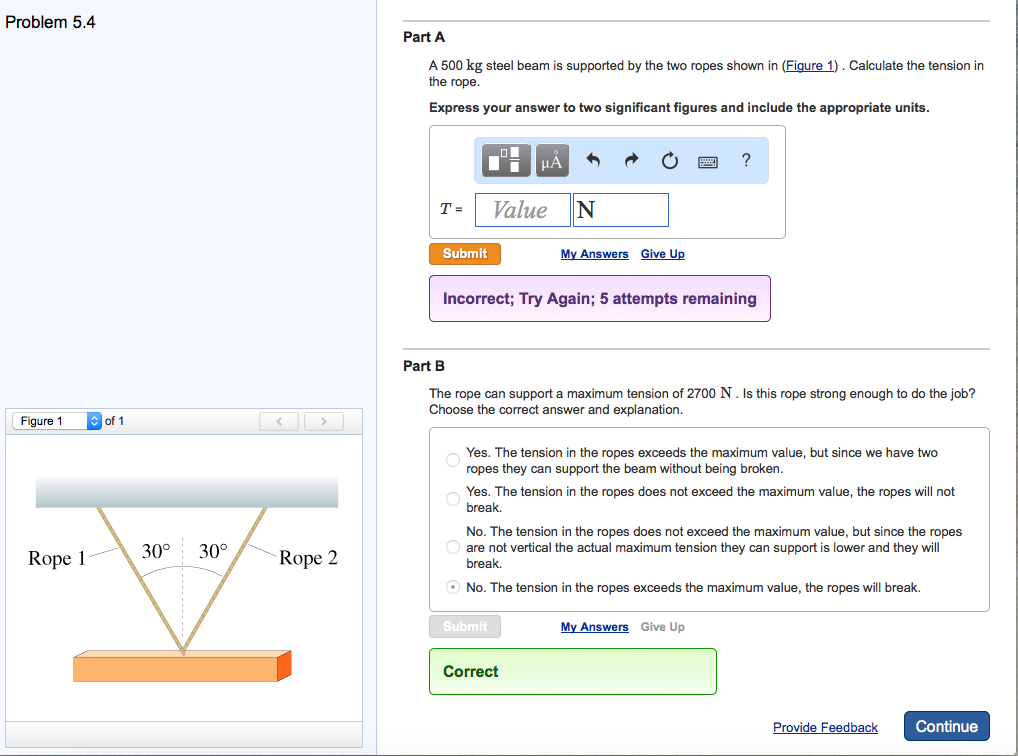Part A A 500 kg steel beam is supported by the two ropes shown in (Figure 1) Calculate the tension in the rope. Express your answer to two significant figures and Include the appropriate units. The rope can support a maximum tension of 2700 N . Is this rope strong enough to do the job? Choose the correct answer and explanation. Yes. The tension in the ropes exceeds the maximum value, but since we have two ropes they can support...

• ### 5. Consider a weight hanging from two wires as in the picture below. a) If 7...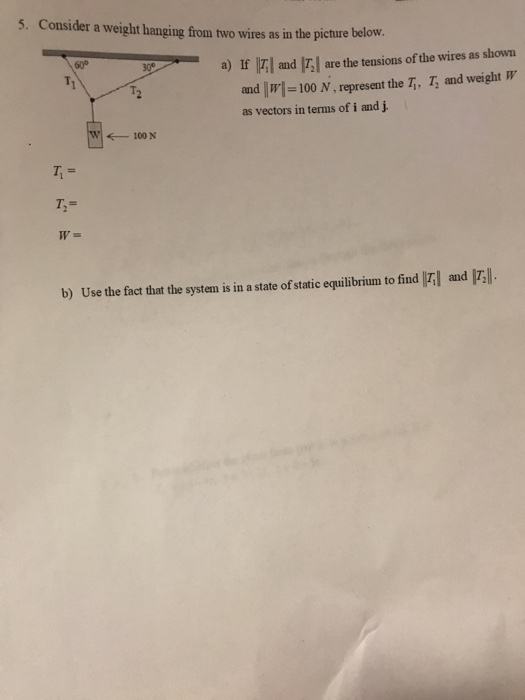5. Consider a weight hanging from two wires as in the picture below. a) If 7 and : are the tensions of the wires as shown and w =100 N , represent the T, T, and weight W as vectors in terms of i and j. W 100 N and 173. b) Use the fact that the system is in a state of static equilibrium to find 7

• ### 1. The three ropes in the figure are tied to a small, very light ring. Two...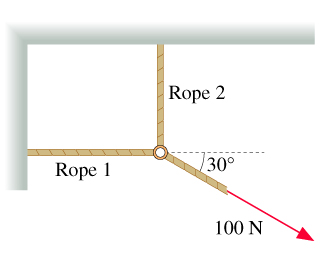1. The three ropes in the figure are tied to a small, very light ring. Two of the ropes are anchored to walls at right angles, and the third rope pulls as shown. What are T1and T2, the magnitudes of the tension forces in the first two ropes? A. T1= B T2= 3. The two angled ropes used to support the crate in the figure below can withstand a maximum tension of 1700N before they break. A. What is the...

Free Homework App